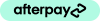•••••••or 4 installments from \$10.25 byMore info
 Color Size Large Lens TypeStrength +0.25+0.50+0.75+1.00+1.25+1.50+1.75+2.00+2.25+2.50+2.75+3.00+3.25+3.50+3.75+4.00+4.25+4.50+4.75+5.00+5.25+5.50+5.75+6.00Clear